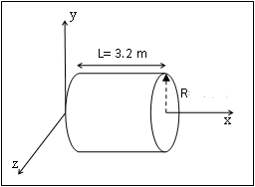# Question Electric field at a certain region of space is given as E⃗=2i^−2j^+2k^ N/C. Calculate the electric flux through the right cap of the cylinder given in the figure if R= 0.6 m ​​​​​​​​​​​​​​&#12304;General guidance&#12305;The answer provided below has been developed in a clear step by step manner.Step1/2Given:electric field, $$\mathrm{{E}={2}\vec{{i}}-{2}\vec{{j}}+{2}\vec{{k}}}$$N/Cradius, R = 0.6 mExplanation:Please refer to solution in this step.Step2/2Explanation:electric flux is defined as: The total number of&#160;electric field&#160;lines passing a given area in a unit t ... See the full answer